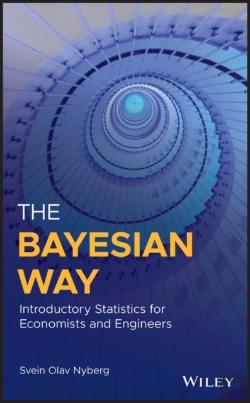# Bayesian Way: Introductory Statistics For Economists And Engineers (eBook)

### de Svein Olav Nyberg

idioma: Inglês
Edição ou reimpressão: julho de 2018
108,52€

A comprehensive resource that offers an introduction to statistics with a Bayesian angle, for students of professional disciplines like engineering and economics The Bayesian Way offers a basic introduction to statistics that emphasizes the Bayesian approach and is designed for use by those studying professional disciplines like engineering and economics. In addition to the Bayesian approach, the author includes the most common techniques of the frequentist approach. Throughout the text, the author covers statistics from a basic to a professional working level along with a practical understanding of the matter at hand. Filled with helpful illustrations, this comprehensive text explores a wide range of topics, starting with descriptive statistics, set theory, and combinatorics. The text then goes on to review fundamental probability theory and Bayes' theorem. The first part ends in an exposition of stochastic variables, exploring discrete, continuous and mixed probability distributions. In the second part, the book looks at statistical inference. Primarily Bayesian, but with the main frequentist techniques included, it covers conjugate priors through the powerful yet simple method of hyperparameters. It then goes on to topics in hypothesis testing (including utility functions), point and interval estimates (including frequentist confidence intervals), and linear regression. This book: Explains basic statistics concepts in accessible terms and uses an abundance of illustrations to enhance visual understanding Has guides for how to calculate the different probability distributions, functions , and statistical properties, on platforms like popular pocket calculators and Mathematica / Wolfram Alpha Includes example-proofs that enable the reader to follow the reasoning Contains assignments at different levels of difficulty from simply filling out the correct formula to the complex multi-step text assignments Offers information on continuous, discrete and mixed probability distributions, hypothesis testing, credible and confidence intervals, and linear regression Written for undergraduate and graduate students of subjects where Bayesian statistics are applied, including engineering, economics, and related fields, The Bayesian Way: With Applications in Engineering and Economics offers a clear understanding of Bayesian statistics that have real-world applications.

Bayesian Way: Introductory Statistics For Economists And Engineers
de Svein Olav Nyberg
ISBN: 9781119246893 Ano de edição ou reimpressão: Editor: WILEY Idioma: Inglês Páginas: 512 Tipo de Produto: eBook Formato: ePUB i Classificação Temática:

### Sugestões

25,90€
(eBook)
FORDHAM UNIVERSITY PRESS
25,90€
(eBook)
FORDHAM UNIVERSITY PRESS
X
O QUE É O CHECKOUT EXPRESSO?

O ‘Checkout Expresso’ utiliza os seus dados habituais (morada e/ou forma de envio, meio de pagamento e dados de faturação) para que a sua compra seja muito mais rápida. Assim, não tem de os indicar de cada vez que fizer uma compra. Em qualquer altura, pode atualizar estes dados na sua ‘Área de Cliente’.

Para que lhe sobre mais tempo para as suas leituras.